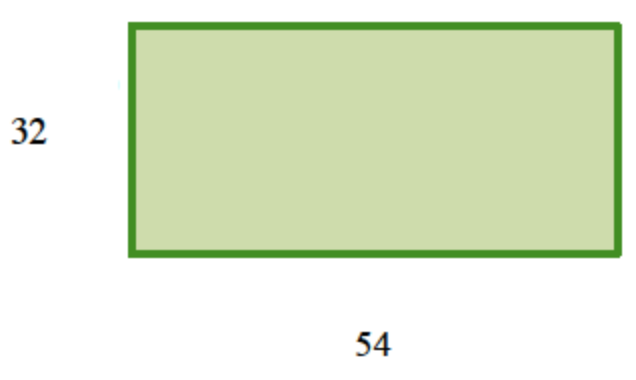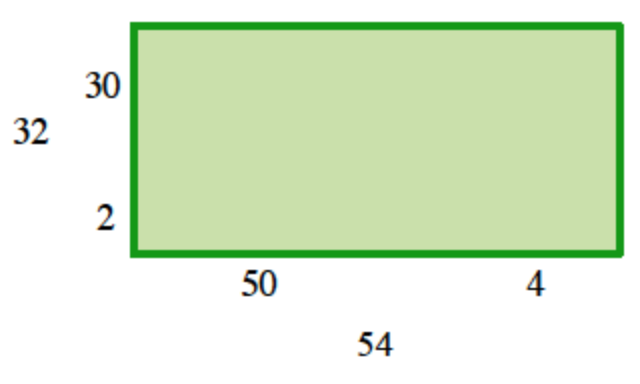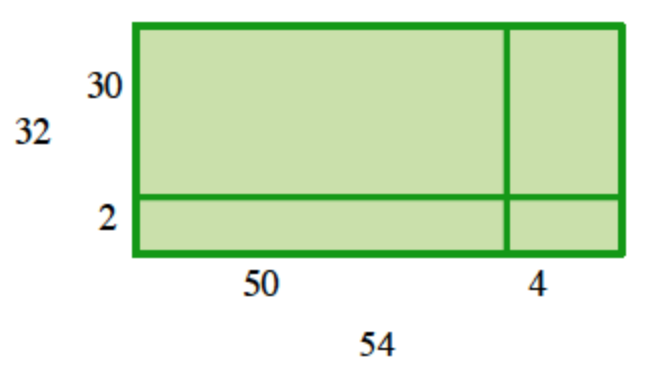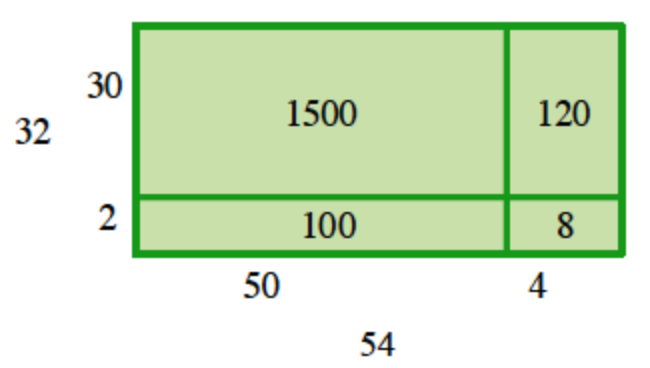### Home > CC2 > Chapter 2 > Lesson 2.2.1 > Problem2-36

2-36.

Read the Math Notes box in this lesson. Then use generic rectangles to complete the following multiplication problems.

1. $54·32$

1. $91·78$

1. $54·32$

Draw a rectangle and label the length and width with the two terms. A horizontal rectangle labeled as follows: bottom edge, 54, left edge, 32.

Do not attempt to draw the rectangle to scale.

Divide each term into easily multiplied smaller terms. Labels added to bottom edge, 50 on the left, and 4 on the right. Labels added to left edge, 30 on top, 2 on bottom.

Subdivide the rectangle so that each smaller term is the dimension of a smaller region of the rectangle. Rectangle is divided into 4 smaller rectangles, 2 rows of 2 columns. Each new rectangle has a label on the bottom and left edge, from the previous picture.

Multiply to find the area of each region. 4 interior rectangles are labeled as follows: top left, 1500, top right, 120, bottom left, 100, bottom right, 8.

If the multiplication is still too difficult, try dividing the rectangle further. For example, $30$ could be written as $10+20$.

Find the product by finding the sum of the smaller areas.

$1500+120+100+8=1728$2. $91·78$

Follow the steps given in part (a).

$7098$